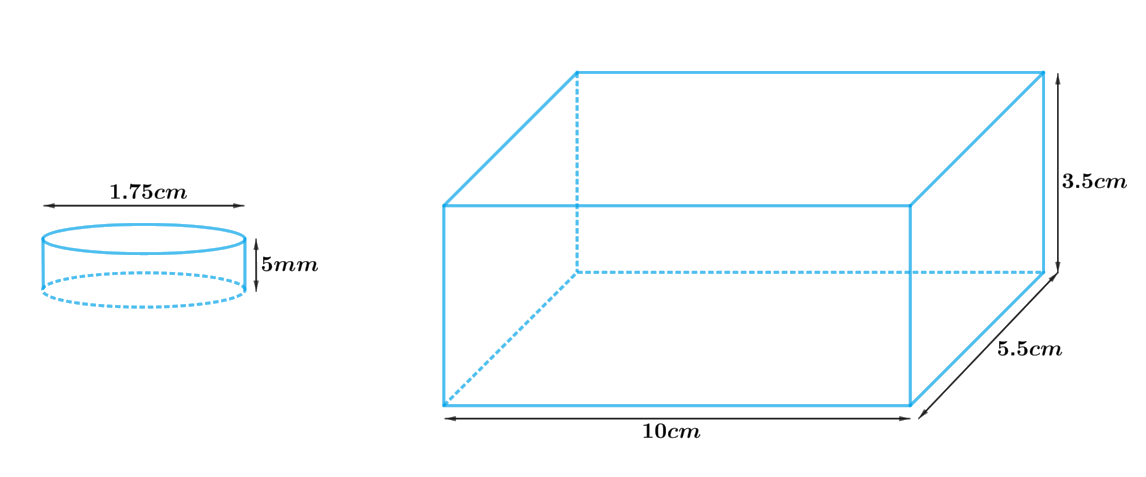# Ex.13.3 Q6 Surface Areas and Volumes Solution - NCERT Maths Class 10

Go back to  'Ex.13.3'

## Question

How many silver coins, $$1.75\, \rm{cm}$$ in diameter and of thickness $$2\, \rm{mm}$$, must be melted to form a cuboid of dimensions  $$5.5\,\rm{cm}$$ $$\times$$ $$10\,\rm{cm}$$ $$\times$$ $$3.5\,\rm{cm}$$?
[Use \begin{align} \pi =\;\frac{22}{7}\end{align}]

Video Solution
Surface Areas And Volumes
Ex 13.3 | Question 6

## Text Solution

What is known?

Diameter of the coin is $$1.75\rm{cm}$$ and thickness is $$2\,\rm{mm.}$$

Dimensions of the cuboid is $$5.5 \rm cm \times 10cm \times 3.5cm$$

What is unknown?

The number of silver coins.

Reasoning:

Draw a figure to visualize the shapes betterFrom the figure it’s clear that the shape of the silver coin is cylindrical.

Since, the silver coins to be melted and recast into a single solid cuboid then the cuboid formed by recasting these coins will be same in volume as the sum of the volumes of these coins.

Sum of the volumes of the silver coins $$=$$ Volume of the cuboid

Therefore,

Number of the coins $$\times$$ volume of each coin $$=$$ volume of the cuboid

We will find the volume of the solid by using formulae;

Volume of the cuboid $$= lbh$$

Where $$l, b$$ and $$h$$ are length, breadth and height of the cuboid respectively.

Volume of the cylinder $$= \pi {r^2}{h_1}$$

where $$r$$ and $$h_1$$ are radius and height of the cylinder respectively.

Steps:

Dimensions of the cuboid,

$$l \times b \times h =\begin{Bmatrix} 5.5 \rm cm \times 10cm \\ \times 3.5 \rm cm \end{Bmatrix}$$

Height of the cylindrical coin,\begin{align} {h_1} = 2mm = \frac{2}{{10}} \rm cm = 0.2{\rm{ }}cm\end{align}

Radius of the cylindrical coin,\begin{align}r = \frac{{1.75 \rm cm}}{2} = {\rm{ }}0.875\, \rm cm\end{align}

Let $$n$$ coins be melted to form the required cuboid.

Volume of $$n$$ coins $$=$$ Volume of cuboids

\begin{align}n \times \pi {r^2}{h_1} &= l \times b \times h\\n &= \frac{{l \times b \times h}}{{\pi {r^2}{h_1}}}\end{align}

\begin{align}&= \frac{{ \begin{bmatrix} 5.5 \rm cm \times 10 \rm cm \times 3.5 \rm cm \end{bmatrix} }}{{ \begin{bmatrix} \frac{{22}}{7} \times {{(0.875 \rm cm)}^2} \times 0.2 \rm cm \end{bmatrix}}}\\&= \frac{{ \begin{bmatrix} 5.5 \rm cm \times 10 \rm cm \times 3.5 \rm cm \end{bmatrix} }}{{ \begin{bmatrix} \frac{{22}}{7} \times {{(0.875 \rm cm)}^2} \times 0.2 \rm cm \end{bmatrix} }}\\&= \frac{{ \begin{bmatrix} 5.5 \rm cm \times 10 \rm cm \times\\ 3.5 \rm cm \times 7 \end{bmatrix} }}{{ \begin{bmatrix} 22 \times 0.875 \rm cm \times 0.875 \rm cm \times \\ 0.2 \rm cm \end{bmatrix} }}\,\, \\&= 400\end{align}

Therefore, the number of coins melted to form such a cuboid is $$400$$.

Learn from the best math teachers and top your exams

• Live one on one classroom and doubt clearing
• Practice worksheets in and after class for conceptual clarity
• Personalized curriculum to keep up with school